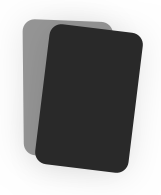# Rational Numbers Unit Flashcards

0.0(0) Reviews
Duplicate
Report Flashcard set

#### Spaced Repetition

Scientifically backed study method#### Flashcards

Review terms and definitions#### Learn

Study with MC, T/F, and other questions#### Practice Test

Take a test on your terms and definitions## Tags

16 Terms
😃 Not studied yet (16)
Absolute Value
The distance a number is away from zero
Distance vs. Change
Distance is always positive To find: Find absolute value of difference (#'s can be in any order) *OR* Subtracting the smaller # from the larger # ____________________________________ Change in elevation/temperature may be pos. or neg. depending on if it's increasing or decreasing (going up or down) Find just like distance, but pos. or neg. answer depending on direction
Commutative Property (addition and multiplication only)
You can swap numbers around and still get the same answer (Only for all adding or all multiplying) **Order does not matter ** Examples: -5 + 3 = 3 + -5 (4)(2) = (2)(4)
Associative Property (addition and multiplication only)
It doesn't matter how we group the numbers (i.e. which we calculate first) You will still get the same answer. (Only for all adding or all multiplying) Examples: (3 + 4) + 5 = 3 + (4 + 5) (3 x 4) x 5 = 3 x (4 x 5)
Distributive Property
The distributive property: we get the same answer when we: added first, then multiply 5(1 + 3) = 5(4)= 20 **OR** multiply each separately then add them 5(1 + 3) = 5(1) + 5(3) = 5 + 15 = 20
Adding zero to a number leaves it unchanged Example: -4 + 0 = -4
Identity Property of Multiplication
Multiplying a number by one leaves the number unchanged Example: 3(1) = 3
What you add to a number to get zero. Example: The additive inverse of -5 is 5 because -5 + 5 = 0. The additive inverse will be the opposite sign.
Inverse Property of Multiplication
"When you multiply a number by its Multiplicative Inverse you get 1. Example: 8 x (1/8) = 1 The multiplicative inverse is also called the reciprocal.
How do you change a decimal to a fraction?
1) Place the digits you see in the numerator 2) The denominator will be the place value that the last digit is in 3) Simplify Example: 0.015 = 15/1000 = 3/200
How do you change a fraction to a decimal?
You can always divide the numerator (top) by the denominator (bottom) **Remember:** numerator goes in the house AND numerator goes in calculator first OR: Change the denominator to a power of ten. (This will not always work)